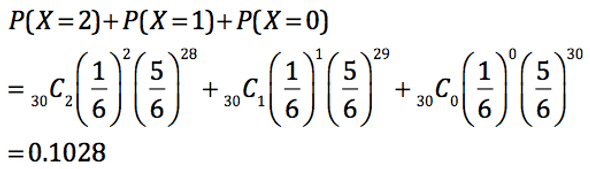top of page

## Chapter 5 - Day 2 - Lesson 5.1

##### Learning Targets
• Use simulation to model chance behavior.

##### Activity: Are soda contests true?

The first strategy (NOT FORMULA!) in this Chapter that students can use to estimate probability is using a simulation. The idea is that if we perform enough trials of a simulation, the proportion of times an event occurs in the long run will approach the true probability (from the Law of Large Numbers in yesterday’s lesson

In this activity, students are trying to find the probability of getting 2 or less winners out of 30, assuming the soda company’s claim is true.

##### Making Connections: Binomial Distribution

In Chapter 6, we will study binomial distributions, where there is a fixed number of trials (n) and a fixed probability of success (p) for each trial. The number of soda bottle winners (X) follows a binomial distribution with n = 30 and p = 1/6. In Chapter 6, we will be able to calculate the exact probability of 2 or less winners:Hopefully your simulation arrives at a good estimate of this probability.

##### Preparing for Inference

We are previewing two very important ideas here:

(1) Conditional probability – Technically this probability is a conditional probability.  It is P(2 or less winners | soda company’s claim is true). Students will see conditional probability formally in Lesson 5.3.

(2) P-value – Not only is this a conditional probability, it is an estimate of the P-value for a significance test. The null hypothesis is that the soda company’s claim is true and we want to figure out how likely it is to get our results (or farther from expected) purely by chance. You may want to plant the seed here for the later interpretation of the P-value and a conclusion for a significance test.

Assuming the soda company’s claim is true, there is a ______ probability of getting 2 or less winners out of 30, purely by chance. This does not provide convincing evidence against the soda company’s claim and is not statistically significant (_____ > 5%). Therefore we fail to reject the null hypothesis and do not have convincing evidence that the soda company is overstating the chances of winning.

bottom of page